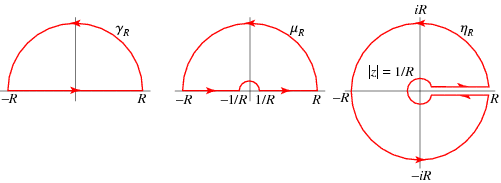# Resiude Question

I have to integrate $\int_{\Gamma} \frac{\log{z+i}}{1+z^2}$ around a large semi-cricular contour.

i found a sinle pole with residue $\frac{\log{2i}}{2i}$ at $z=i$ and a double pole with residue $-\frac{1}{2i}-\frac{\log{2i}}{2i}$ at $z=-i$. this seems fair enough but i can't decide where to put my contour so as to enclose them both. the reside theorem tells me they will integrate to $-\pi$ if i can get them both inside the contour which is probably a good aim consdiering the next part of the question is to show

$\int_0^{\infty} \frac{\log{x^2+1}}{x^2+1}dx=\pi \log{2}$

so
(i) where do i put the contour
(ii) how can i evaluate the next part given that my complex integral is in terms of z's and the one i want is in terms of x^2's so it isn't as simple as taking the real part after i use Jordan's lemma on teh semi circle is it?

thanks

Can you double check that wrote down the problem down correctly, both the first and second parts? Things just seem a little off, maybe not though.

There are a few things.
1) Why is the point z=-i a pole of order 2 and not 1?
2) The countour you will need is $\Gamma = \gamma_R + [-R,-1/R] +\gamma_{1/R} + [r,R]$, where $\gamma_R = Re^{it},\,\gamma_{1/R} = -e^{-it}/R$ and $R>0,\,t\in[0,\pi]$. You'll let $R\to\infty$, and note that $1/R\to0$. Look at the second contour in the below figure.You need such a contour because you need $\log z$ to be an analytic branch of the logarithm, for example the branch that deletes 0 and the negative imaginary axis.
3) The way to do such an integral is:
\begin{align*} \lim_{R\to\infty} \int_\Gamma f(z) \,dz &= \lim_{R\to\infty} \left( \int_{\gamma_R} f(z)\,dz + \int_{-R}^{-1/R} f(x)\,dx + \int_{\gamma_{1/R}} f(z) \,dz + \int_{1/R}^R f(x)\,dx \right) \\ 2\pi i \sum_{z_k\in \Gamma} \text{Res}\, (f;z_k) &= \int_{-\infty}^0 f(x)\,dx + \int_0^\infty f(x)\,dx + \lim_{R\to\infty} \left( \int_{\gamma_R} f(z)\,dz + \int_{\gamma_{1/R}} f(z) \,dz \right) \\ \end{align*}
The integrals left over on the right are usually shown to tend to zero as $R\to\infty$ or are evaluated otherwise.
4) You don't need all the poles of f(z) to be inside $\Gamma$. You just worry about the ones that are as $R\to\infty$.
5) Also, if f(x) is even then
$$\int_{-\infty}^0 f(x)\,dx + \int_0^\infty f(x) \,dx = \int_{-\infty}^\infty f(x) \,dx = 2\int_0^\infty f(x) \,dx$$

#### Attachments

it is meant to be log(z+i) and log(x^2+i)

you say we need log to be holomorphic so we deleted zer but our contour still contains the negative real axis?

with such a contour, i would only have the simple pole's contribution

i said we had a double pole at z=-i because initially when i went for a simple pole and started evaluating the residues, i got something divided by 0 (which usually means you've missed an order of your pole doesn't it?) so i assumed that log wouldn't be holomorphic at z=-i either and that made it a double pole and the residue calculation then worked fine

can you comment on these three points please